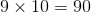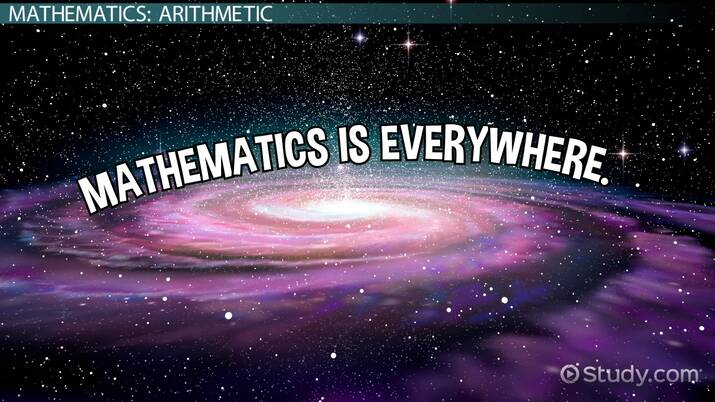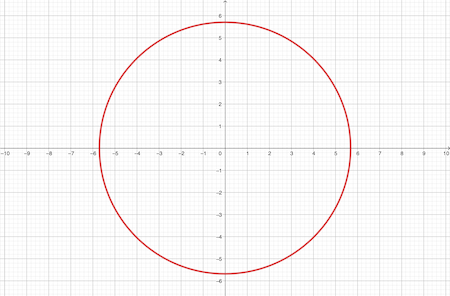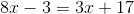TExES Mathematics/Science 4-8 (114) Test Prep / Course / Chapter

# Type of Mathematics with Examples

Katherine Kaylegian-Starkey, Usha Bhakuni
• Author
Katherine Kaylegian-Starkey

Katherine has a bachelor's degree in physics, and she is pursuing a master's degree in applied physics. She currently teaches struggling STEM students at Lane Community College.

• Instructor
Usha Bhakuni

Usha has taught high school level Math and has master's degree in Finance

The goal of this lesson is to understand the field of mathematics and the different types of mathematics that are used to explain the world. Updated: 12/11/2021

Show

#### What is math in our life?

Math is found in daily life. Balancing a checkbook, measuring out a recipe, and counting paper plates for a party are all examples of mathematics in daily life. Many professionals also use math daily at work such as engineers, businessmen, architects, contracts, and scientists.

#### What exactly is math?

Math is a numerical study of the world. It is a field of science that quantifies and describes the world using numbers.

Mathematics is a numerical study of the world. It is a branch of science that is concerned with numerical values, quantities, amounts, shapes, and spaces. Mathematics often relates numerical values, shapes, spaces, and quantities through formulas. The ancient Greek mathematician and inventor Archimedes studied such things as water and shapes, and he is credited with being the father of mathematics.

To unlock this lesson you must be a Study.com Member.

## Mathematics: Arithmetic

Mathematics is one of the most important areas of study for mankind. It has applications in almost all fields: science, technology, business, medicine, meteorology, astronomy, etc. From elementary addition and subtraction to rocket science, mathematics is present everywhere.

Mathematics is a vast area of study and has developed throughout history. It's divided into several disciplines. Let's take a look at some of the popular disciplines.

Arithmetic is one of the oldest disciplines of mathematics and also the most basic. It deals with numbers and elementary operators (addition, subtraction, multiplication, and division). All the basic rules of interactions of these operators with numbers are covered in arithmetic. You can say that arithmetic is everyday math, useful for handy calculations. A simple multiplication problem is:These numbers and operators form the basic building blocks of other disciplines of mathematics.An error occurred trying to load this video.

Try refreshing the page, or contact customer support.

Coming up next: Integrating Math & Other Subjects

### You're on a roll. Keep up the good work!

Replay
Your next lesson will play in 10 seconds
• 0:04 Mathematics: Arithmetic
• 1:09 Algebra & Geometry
• 2:20 Trigonometry & Calculus
• 3:22 Statistics & Probability
• 4:18 Lesson Summary
Save Timeline
Autoplay
Autoplay
Speed Speed

There are many types of mathematics because quantifying the world can be broken into many categories, and, over the years, each category has developed its own branch. For example, the study of rates of change has its own branch of math called calculus. The study of circles and right triangles and how they relate is called trigonometry, and the study of all shapes is called geometry. There are many more types of math other than these three, and the types of math described below are only a part of the expansive field of mathematics.

### Arithmetic

Arithmetic is the study of numbers. It can be considered the basis of all mathematics, and the field of arithmetic consists of concepts such as counting and the manipulation of counting numbers. Addition, subtraction, multiplication, division, and exponentiation are all examples of arithmetic. Arithmetic is the most commonly used form of mathematics. Arithmetic is used when balancing a checking account, paying for items, or adding up the boxes of Girl Scout Cookies sold. Arithmetic is also used in physics, chemistry, biology, business, and any other field that uses numbers.

Examples of Arithmetic
a) Add 5 and 3. b) Multiply 2 and 4.

a) Adding means taking a group of 5 and a group of 3 and combining them. In math, addition uses a + symbol so 5 plus 3 is written as 5 + 3 and 5 + 3 = 8.

b) Multiplying means adding multiple groups of a number. This example asks that 4 groups of 2 be added. Multiplication can be written using an x or an *. In this example, 2*4 = 8.

### Algebra

Many times, when trying to describe the world, some quantities are known but one or more quantities are unknown. The field of algebra is dedicated to the study of unknown numbers which are called variables. Algebra is also associated with functions which are mathematic expressions that define a relationship, and functions always contain at least one unknown quantity. The function {eq}r(x, y) = \sqrt(x^2 + y^2) {/eq} relates two points, x and y, and to the radius of a circle, r. This function, depicted in Figure 1, is the algebraic way to describe a circle.Example of Algebra
Find the value of the variable in the expression 3 - x = 10.

Conceptually, this example wants to know what number when subtracted from 3 will equal 10. To solve this expression, the goal is to get the variable alone on one side. This can be done by subtracting 3 from both sides so that the expression becomes 3 - x - 3 = 10 -3. The 3's cancel on the left, and all that is left is -x = 7. Convention says that the variable should be positive, so x = -7. When -7 is subtracted from 3 it does indeed give a final answer of 10.

### Geometry

Geometry is one of the oldest forms of mathematics, and it was Archimedes who was the first to calculate the value of {eq}\pi {/eq}, a naturally occurring value that is crucial to mathematics. Geometry concerns itself with the measurement of shapes, and the concepts of area, surface area, volume, and perimeter belong to the field of geometry. Geometry is used in physics, chemistry, biophysics, engineering, and other fields that are concerned with shapes.

Example of Geometry
Find the perimeter of the square in Figure 2.

To unlock this lesson you must be a Study.com Member.

Mathematics is a branch of science that numerically studies the world. Mathematics concerns itself with numerical values, formulas, quantities, amounts, and shapes. Archimedes is credited with being the father of mathematics. He was the first to calculate {eq}\pi {/eq} and the first to numerically describe many shapes such as spheres. The study of shapes, called geometry, is one of the oldest branches of mathematics.

There are many branches of mathematics that have appeared since the beginning. Arithmetic, like geometry, was created by Archimedes, and since then algebra, trigonometry, calculus, and statistics have emerged. Each type of math came about as a way to study a specific attribute of the world, and, in practice, most fields combine multiple types of math.

To unlock this lesson you must be a Study.com Member.

## Algebra & Geometry

One of the most widely used disciplines of mathematics, algebra involves the study of mathematical variables, functions, equations, and the rules associated with them. It uses unknown quantities represented by letters or symbols. It's the most basic and essential field of study and has applications across all the disciplines. Most commonly, it's used for solving equations for unknown variables. A typical algebra problem would look like:The equation-solving rules in algebra are often applied in other disciplines as well.

The study of shapes, sizes, distance, location, etc. is called geometry. It covers length, area and volume, in one-, two-, and three-dimensional planes, respectively. It has many applications in our day-to-day life, such as finding the area of a plot of land, finding volume of a gas cylinder, etc. It also has many complex applications, such as in the field of astronomy to mark locations of stars, calculating distances between two celestial bodies, etc.

## Trigonometry & Calculus

Trigonometry involves the study of the relationship between angles and sides of a triangle. Most commonly it is used for right-angled triangles. It has special functions, called trigonometric functions, that relate the sides and angles. In real life applications, it's used commonly for finding heights of towers, buildings, mountains, etc. Trigonometric functions are also used in study of waves and their characteristics.

Video Transcript

## Mathematics: Arithmetic

Mathematics is one of the most important areas of study for mankind. It has applications in almost all fields: science, technology, business, medicine, meteorology, astronomy, etc. From elementary addition and subtraction to rocket science, mathematics is present everywhere.

Mathematics is a vast area of study and has developed throughout history. It's divided into several disciplines. Let's take a look at some of the popular disciplines.

Arithmetic is one of the oldest disciplines of mathematics and also the most basic. It deals with numbers and elementary operators (addition, subtraction, multiplication, and division). All the basic rules of interactions of these operators with numbers are covered in arithmetic. You can say that arithmetic is everyday math, useful for handy calculations. A simple multiplication problem is:These numbers and operators form the basic building blocks of other disciplines of mathematics.

## Algebra & Geometry

One of the most widely used disciplines of mathematics, algebra involves the study of mathematical variables, functions, equations, and the rules associated with them. It uses unknown quantities represented by letters or symbols. It's the most basic and essential field of study and has applications across all the disciplines. Most commonly, it's used for solving equations for unknown variables. A typical algebra problem would look like:The equation-solving rules in algebra are often applied in other disciplines as well.

The study of shapes, sizes, distance, location, etc. is called geometry. It covers length, area and volume, in one-, two-, and three-dimensional planes, respectively. It has many applications in our day-to-day life, such as finding the area of a plot of land, finding volume of a gas cylinder, etc. It also has many complex applications, such as in the field of astronomy to mark locations of stars, calculating distances between two celestial bodies, etc.

## Trigonometry & Calculus

Trigonometry involves the study of the relationship between angles and sides of a triangle. Most commonly it is used for right-angled triangles. It has special functions, called trigonometric functions, that relate the sides and angles. In real life applications, it's used commonly for finding heights of towers, buildings, mountains, etc. Trigonometric functions are also used in study of waves and their characteristics.

To unlock this lesson you must be a Study.com Member.

### Register to view this lesson

Are you a student or a teacher?

Back

### Resources created by teachers for teachers

Over 30,000 video lessons & teaching resources‐all in one place.Video lessonsQuizzes & WorksheetsClassroom IntegrationLesson Plans

I would definitely recommend Study.com to my colleagues. It’s like a teacher waved a magic wand and did the work for me. I feel like it’s a lifeline.

Jennifer B.
TeacherCreate an account to start this course today
Used by over 30 million students worldwide